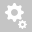##Recently viewed tickets# Deflections during Construction Staging Analysis

Question:

Can you please provide confirmation as to how Midas treats deflections of multiple segments during a construction staging analysis? I have this option on in my modelHi, Thanks for writing to us.

Understanding deformation behavior in Construction Stage Analysis:

Example problem: Consider a cantilever that will be cast in three parts.

To simulate the above construction stages in Midas, we have created three stages as shown below CS1, CS2, CS3 (green dashed line indicates the construction joint).

Deformation results in each CS stage after analysis are as below:

1) Construction stage deformation  (CS deformation)
2) Current Step Displ.
3) Stage/Step Real Displ.Let us dissect how Midas calculates the deformation results in each CS stage:

CS1 --> CS1 deformation = (current step Displ. of CS1)
CS2 --> CS2 deformation = (CS1 deformation)  + (current step Displ. of CS2)
CS3 --> CS3 deformation = (CS2 deformation)  + (current step Displ. of CS3)

CS1 --> CS1 Stage/Step Real Displ. = (current step Displ. of CS1) + (Tangent displacements)
CS2 --> CS2 Stage/Step Real Displ. = (CS1 deformation)  + (current step Displ. of CS2) + (Tangent displacements)
CS3 --> CS3 Stage/Step Real Displ. = (CS2 deformation)  + (current step Displ. of CS3) + (Tangent displacements)

Based on the above formulas, we can validate the CS2 deformation = (CS1 deformation)  + (current step Displ. of CS2); by preparing another model which has the below load pattern and then running a simple static analysis.

Adding above to displacements gives us the below displacements. CS2 stage deformation = [(CS1 deformation)  + (current step Displ. of CS2)]

where as Stage/Step Real Displ. values are below (where tangent displacements are additionally added).

PFA the verification models.

Thanks,
MIDAS TECH SUPPORT

Creation date: 6/25/2020 2:59 AM (suman)      Updated: 6/25/2020 12:45 PM (suman)